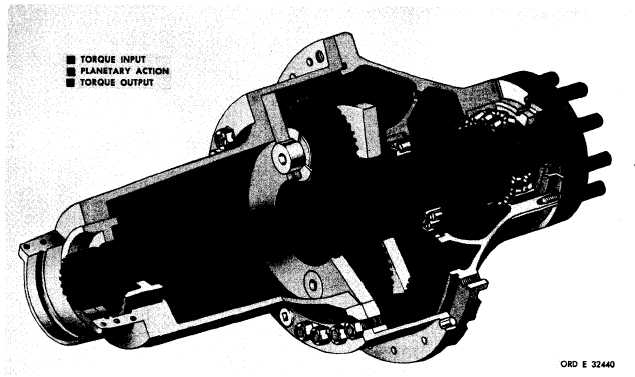Custom SearchFigure 21 C H A P    2,   SEC   V T O R Q U E     P A T H S P A R   4  5 Figure  21.  Output  drive  torque  path 45. TORQUE PATH - OUTPUT DRIVES ( f i g .    2 1) .   F i g u r e    2 1    i s    a    c u t a w a y    v i e w    o f    t h e left-output      drive   (mounts   at   right   side   of   power train).   The   construction   of   the   right   assembly i s     i d e n t i c a l     e x c e p t     f o r     l e n g t h ;     o p e r a t i o n     i s i d e n t i c a l. .   T o r q u e    i s    t r a n s m i t t e d    f r o m    t h e    m a in transmission   section   output   to   the   output   drive assembly   by   the   output   drive   sun   gear   shaft. The   integral   sun   gear   imparts   torque   to   the planetary   pinions   which   are   mounted   in   a   sta- tionary   carrier.   In   turn,   the   pinions   transmit torque   to   the   ring   gear.   The   ring   gear,   splined to   the   output   shaft,   transmits   torque   to   the output   shaft. .   Arrows   indicating   the   direction   of   ro- t a t i o n    i l l u s t r a t e    c o n d i t i o n s    w h e n    t h e    p o w er train   is   operating   in   a   forward   gear.   In   a   re- verse   gear,   all   rotations   would   reverse.   Note that   the   output   drive   output   rotation   is   opposite that   of   the   output   drive   input   because   the   re- a c t i o n    m e m b e r    ( s t a t i o n a r y    m e m b e r )    i s    t he p l a n e t a r y     c a r r i e r. 4 8